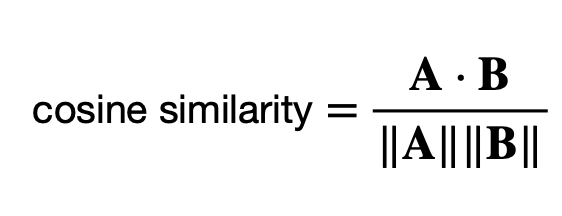## Pages

### Cosine Similarity Computing Example with Scikit-learn

Cosine similarity is a useful metric in various fields, including natural language processing, information retrieval, recommendation systems, and more. Its primary purpose is to measure the similarity or dissimilarity between two non-zero vectors in a multi-dimensional space, and it serves several important purposes. In the context of text data, it's often used to measure the similarity between two documents or sentences.

In this blog post, we'll delve into cosine similarity and its applications with Scikit-learn API. The tutorial covers:
1. The concept of cosine similarity
2. Computing cosine similarity
3. Conclusion

Let's get started.

The concept of cosine similarity

Cosine similarity is a metric used to measure how similar two non-zero vectors are in a multi-dimensional space. It's often employed in various fields, including natural language processing, document retrieval, and recommendation systems. Cosine similarity quantifies the cosine of the angle between two vectors, and it ranges from -1 (completely dissimilar) to 1 (completely similar), with 0 indicating no similarity.

Here's how cosine similarity works in the context of text data, which is a common application:

Vector Representation: Documents or sentences are represented as vectors in a multi-dimensional space. Each dimension typically corresponds to a word or a term, and the value of each dimension represents the importance or frequency of that word in the document. One common vectorization technique is TF-IDF (Term Frequency-Inverse Document Frequency).

Cosine of the Angle: The cosine similarity between two vectors is computed by taking the dot product of the vectors and dividing it by the product of their magnitudes (lengths). Mathematically, it's defined as:Where:
A ⋅ B is the dot product of vectors A and B.
||A|| and ||B|| are the magnitudes (lengths) of vectors A and B.

Interpreting the Result: If the vectors are identical (point in the same direction), the cosine similarity is 1, indicating they are perfectly similar. If the vectors are orthogonal (at a 90-degree angle), the cosine similarity is 0, indicating no similarity. If the vectors are diametrically opposed (point in opposite directions), the cosine similarity is -1, indicating they are completely dissimilar.

Computing cosine similarity

Scikit-learn provides a function for computing cosine_similarity.  In below example, we'll compute the cosine similarity for given text by using scikit-learn. First, we'll define sample phrases to check the similarity. We use the TfidfVectorizer to convert the sample phrases and given text into TF-IDF (Term Frequency-Inverse Document Frequency) vectors. The TF-IDF vectors are then used to calculate cosine similarity between the sample phrases and input phrase using cosine_similarity from scikit-learn's metrics.pairwise module. Finally, we'll print the cosine similarity scores for each standard phrases.

from sklearn.feature_extraction.text import TfidfVectorizer
from sklearn.metrics.pairwise import cosine_similarity

# Sample phrases
standard_phrases = [
"Code efficiently",
"Debug with patience",
"Think logically",
]

# Create a TF-IDF vectorizer
tfidf_vectorizer = TfidfVectorizer()

# Fit and transform the standard phrases
tfidf_standard_phrases = tfidf_vectorizer.fit_transform(standard_phrases)

# Define a new input phrase
input_phrase = "Code can be beautiful."

# Transform the input phrase
tfidf_input = tfidf_vectorizer.transform([input_phrase])

# Compute cosine similarity between the input phrase and
# each standard phrase
cosine_sim_scores = cosine_similarity(tfidf_input, tfidf_standard_phrases)
# Print the cosine similarity scores for each standard phrase
for ph, score in zip(standard_phrases, cosine_sim_scores):
print(f"Similarity between '{input_phrase}' and '{ph}': {score:.4f}")
` `

And result looks as below.

Similarity between 'Code can be beautiful' and 'Code efficiently': 0.7071
Similarity between 'Code can be beautiful' and 'Debug with patience': 0.0000
Similarity between 'Code can be beautiful' and 'Automate task': 0.0000
Similarity between 'Code can be beautiful' and 'Think logically': 0.0000

Conclusion

In this tutorial, we've briefly explored the concepts of cosine similarity and learned how to implement it with scikit-learn.
Cosine similarity is a versatile metric that helps quantify the degree of similarity between data points or documents, making it valuable in a wide range of applications for measuring and understanding similarity relationships in multi-dimensional spaces.

References: